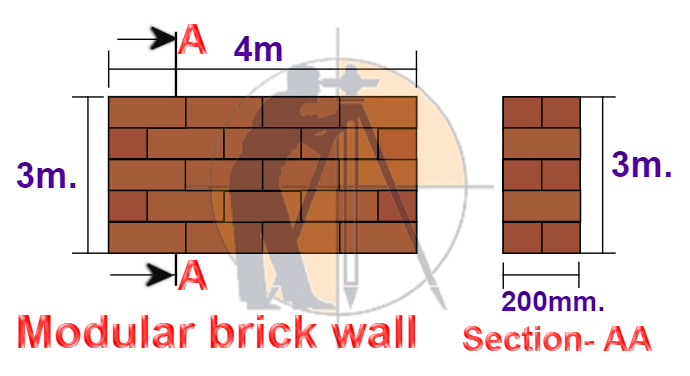Breaking News
Home / QA/QC Engineering / How To Calculate The Cost Of Modular Brick Wall Masonry With Full Detail

# How To Calculate The Cost Of Modular Brick Wall Masonry With Full Detail

## How To Calculate The Cost Of Modular Brick Wall Masonry With Full Detail

Let us consider modular brick wall masonry as shown in the drawing for calculation purposes.### Given data:

Length of the brick wall = 4m.

Height of the brick wall = 3m.

The thickness of the brick wall = 200mm. = 0.20m.

### The total volume of the brick wall masonry

= length × height × thickness

= 4m. × 3m. × 0.20m..

### = 2.40 cum.

The total area of brick wall

= length × height

=4m. × 3m.

### A.  Material calculation:

No. of bricks required/ cum. of wall = 500 nos.

No. of Cement bags required/ cum. of wall = 1.261 bags

The volume of sand required/ cum. of wall = 0.2628 cum.

### Note :

The requirement of bricks, cement & sand per cum. is taken from the above-mentioned article.

1. ### No. of  bricks required

= [vol. of brick wall × bricks/ cum.]

=  [2.4 cum. × 500 nos.]

1. ### No. of  cement bags required

= [vol. of brick wall × cement / cum.]

=  [2.4 cum. × 1.261bags]

1. ### The volume of sand required

= [vol. of brick wall × sand/ cum.]

=  [2.4 cum. × 0.2628 cum.]

### The total cost of labor for modular brick masonry

= total area of brick masonry in sqm.  × labor rate/ sqm.

= 12 sqm. × 170/-

1. ### The cost of modular brick wall masonry/sqm.

= [total brick masonry cost ÷ total brick wall area]

1. ### The cost of modular brick wall masonry/cum.

= [total brick masonry cost ÷ total vol. of the brick wall]

= 13,851.98 ÷ 2.4

1. ### The cost of modular brick wall masonry/sq ft.

= [1154.33 ÷ 10.764]

1. ### The cost of modular brick wall masonry/cu ft.

[5771.66 ÷ 35.3147]

### THANKS.